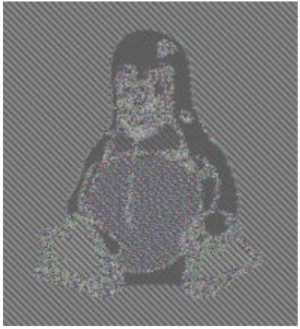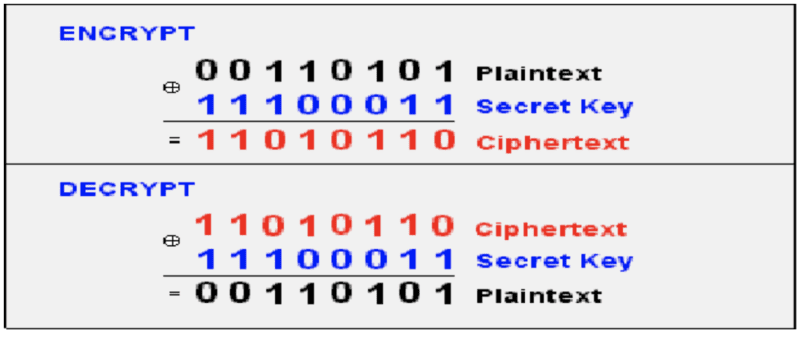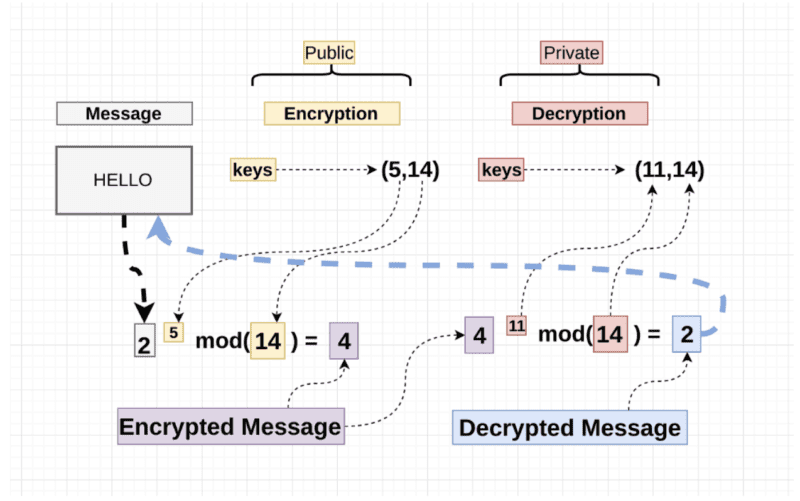# Types of Encryption Algorithms + Pros and Cons for Each

Types of Encryption Algorithms + Pros and Cons for Each

Different types of encryption algorithms are designed to obfuscate data in a way that it is only possible to extract the original data with the correct secret key. However, there are a variety of different ways of accomplishing this.

The two main categories of encryption algorithms are symmetric and asymmetric encryption. Each of these approaches to encryption has its pros and cons.

## Symmetric Encryption

Symmetric encryption algorithms use the same secret key for both encryption and decryption. This means that the sender and the recipient of an encrypted message need to share a copy of the secret key via a secure channel before starting to send encrypted data. Symmetric encryption algorithms come in two different varieties: block and stream ciphers.

### Block Ciphers

A block cipher encrypts data in fixed-size chunks. For example, the Advanced Encryption Standard (AES) uses a block length of 128 bits.

If the plaintext is shorter than the block length, then it is padded out to the desired length before encryption. At the other end, the recipient of the message will decrypt it and then remove the padding to restore the original message.

If a plaintext is longer than the block length, then it is broken up into multiple different chunks for encryption. A block cipher mode of operation defines how these chunks are related to one another.

Each mode of operation has its pros and cons. For example, Electronic Code Book (ECB) mode is the simplest mode of operation. With ECB, each block is encrypted completely independently.The downside of this is that blocks with the same plaintext produce the same ciphertext.  The image above is a picture of the Linux penguin. While this data is encrypted, the ciphertexts for a pixel of a certain color (black, white, etc.) are the same throughout the image, so the penguin is still visible.

Other modes of operation eliminate this issue by interrelating the encryption of each block.  Some also provide additional features, such as Galois Counter Mode (GCM), which generates a message authentication code (MAC) that verifies that data has not been modified in transit.

#### Example: The Advanced Encryption Standard (AES)

The most famous block cipher is the Advanced Encryption Standard (AES). This encryption algorithm was selected as the result of a contest run by the National Institute of Standards and Technology (NIST) to replace the aging Data Encryption Standard (DES).

AES is a family of three different algorithms designed to use a 128, 192, or 256 bit encryption key. These algorithms are broken into a key schedule and an encryption algorithm.

The encryption algorithm of AES is largely the same for all three versions.  It is divided into rounds, which are composed of a set of mathematical operations. The main difference between the different AES versions is the number of rounds used: 10, 12, and 14.

Each round of AES uses a unique round key that is derived from the original secret key.  Deriving these round keys is the job of the key schedule  Each AES version’s key schedule is different because they take different length secret keys and produce different numbers of 128-bit round keys.

### Stream Ciphers

The other type of symmetric encryption algorithm is a stream cipher. Unlike a block cipher, a stream cipher encrypts a plaintext one bit at a time.A stream cipher is designed based on the only completely unbreakable encryption algorithm: the one-time pad (OTP). The OTP takes a random secret key the same length as the plaintext and exclusive-ors (XORs) each bit of the plaintext and key together to produce the ciphertext as shown in the image above.

Decryption with a OTP is the same as encryption. This is because anything XORed with itself is zero and anything XORed with zero is itself.  With a plaintext P, ciphertext C, and key K

C XOR K = (C XOR K) XOR K = C XOR (K XOR K) = C XOR 0 = C

While it has great security, the OTP is rarely used because it is impractical to securely share the massive amounts of key material that it needs to work. A stream cipher uses the same idea as the OTP with a slightly less secure key.

Instead of a fully random key, a stream cipher uses a secret key to feed a pseudo-random number generator. By sharing the same secret key and algorithm, the sender and recipient of a message can crank out the same string of bits, enabling them to encrypt and decrypt a message.

#### Example: Rivest Cipher 4 (RC4)

RC4 is an example of a widely-used stream cipher. It was created by Ron Rivest in 1987 and was originally a trade secret of RSA Security. In 1994, the details of the cipher were leaked, making it publicly usable.

RC4 is used in a variety of different applications, including the WEP and WPA encryption standards for Wi-Fi. The cipher has some known vulnerabilities, especially for certain applications, but can still be used if some of the initial bytes of the generated keystream are discarded.

## Asymmetric Encryption

Unlike symmetric encryption, asymmetric cryptography uses two different keys for encryption and decryption. The public key is used to encrypt a message, while the private key is used for decryption.

The private key is a completely random number. The public key is derived from the private key using a mathematically “hard” problem.

This “hard” problem is based on a mathematical operation that is “easy” to perform but “hard” to reverse. A number of different “hard” problems are used, including integer-based ones and ones based upon elliptic curves.

### Integer-Based Cryptography

Integer-based asymmetric cryptography uses two main “hard” problems. These are the factoring and discrete logarithm problems.

The factoring problem is based on the fact that it is relatively easy to multiply two numbers together but it is hard to factor them. In fact, factoring is so hard that the best way to do so (on a “classical” computer) is through a brute force search. Someone wanting to factor the product of two prime numbers needs to test potential factors until they happen to find one of the two factors, which can take a very long time.

An asymmetric encryption algorithm based on the factoring problem will have a public key calculated using the product of two private keys (large prime numbers). This calculation is easy to perform, but anyone wanting to derive the private key from the public key will need to factor it, which is much harder.

The difficulty of multiplication grows polynomially with the length of the factors, but the difficulty of factoring grows exponentially. This makes it possible to find a “sweet spot”, where a system is usable but essentially unbreakable.

The discrete logarithm problem uses exponentiation and logarithms as its “easy” and “hard” operations. Similar to factoring, the complexity of calculating logarithms grows much more quickly as the size of the exponent increases.

#### Example: Rivest-Shamir-Adleman (RSA)

Symmetric encryption is a simple cryptographic algorithm by today’s standards, however, it was once considered state of the art. In fact, the German army used it to send private communications during World War II. The movie The Imitation Game actually does quite a good job of explaining how symmetric encryption works and the role it played during the war.

With symmetric encryption, a message that gets typed in plain text goes through mathematical permutations to become encrypted. The encrypted message is difficult to break because the same plain text letter does not always come out the same in the encrypted message. For example, the message “HHH” would not encrypt to three of the same characters.

To both encrypt and decrypt the message, you need the same key, hence the name symmetric encryption. While decrypting messages is exceedingly difficult without the key, the fact that the same key must be used to encrypt and decrypt the message carries significant risk. That’s because if the distribution channel used to share the key gets compromised, the whole system for secure messages is broken.

One of the most famous asymmetric encryption algorithms in existence is the one developed by Ron Rivest, Adi Shamir, and Leonard Adleman called RSA. This algorithm is based on the factoring problem.The image above shows a simple example of how RSA works. The plaintext (2) is raised to the power of the public key (5): 2^5 = 32. This value is then divided by a public modulus (14) and the remainder (4) is sent as the ciphertext: 32 % 14 = 4.

At the other end, the same operation is performed with the private key instead of the public key to produce the plaintext: (4^11) % 14 = 2. This calculation works because the public and private keys are selected so that they are inverses in the chosen modulus.

### Elliptic-Curve Cryptography

Integer-based asymmetric cryptography uses factoring and discrete logarithm problems to build secure encryption algorithms. Elliptic curve cryptography uses the same problems with a little twist.

Instead of using integers for its calculations, elliptic curve cryptography uses points on an elliptic curve, like the one shown above. A private key is still a random number, but a public key is a point on the curve.

A few different mathematical operations are defined on these curves. The two important ones here are:

• Point Addition (equivalent to integer multiplication)
• Point Multiplication (equivalent to integer exponentiation)

On these curves, it is possible to perform calculations that are equivalent to the “easy” operations of the factoring and discrete logarithm problems. This means that the same basic algorithms can be adopted to use with elliptic curves.

But why bother? Elliptic curve cryptography is useful because smaller key lengths provide the same level of security. This means that elliptic curve cryptography uses less storage, processing power, and energy to protect data at the same level as an equivalent integer-based algorithm. These savings can be important for resource-constrained systems like Internet of Things (IoT) devices or smartphones.

## Pros and Cons of Symmetric and Asymmetric Encryption

Symmetric and asymmetric encryption algorithms both are designed to do the same job: protecting the confidentiality of data. However, they do their jobs in very different ways, and each approach has its pros and cons:

• Symmetric Encryption: The main advantage of symmetric cryptography is its efficiency.  In general, symmetric encryption algorithms use less memory and processing power than asymmetric cryptography.
• Asymmetric Encryption: Asymmetric encryption does not require the two parties to securely share a secret key before sending encrypted messages.  This makes it possible to securely communicate with anyone as long as you have their private key.

These different strengths mean that symmetric and asymmetric cryptography are often used together, like in the TLS protocol. Asymmetric encryption is used to securely exchange a symmetric key, and symmetric encryption is used for bulk data transfer.

### Quantum Computing and Its Impacts on Cryptography

When discussing the pros and cons of different encryption algorithms, it is important to take into account the growth of quantum computing. Quantum computers have the ability to break some of the asymmetric encryption algorithms in common use today.

The reason for this is that some of the “hard” problems used in asymmetric cryptography are not “hard” for quantum computers. While factoring is exponentially difficult for a classical computer, it only has polynomial difficulty for a quantum computer due to the existence of Shor’s algorithm.

If multiplication and factoring both have polynomial complexity, then it is impossible to build a cryptosystem using this problem that is both usable and secure. The same is true for the discrete logarithm problem. It is also broken once large enough quantum computers become available.

However, this does not mean that quantum computing will be the end of asymmetric cryptography. New problems have been discovered that are believed to be “hard” for quantum computers as well. This has led to the development of new post-quantum asymmetric encryption algorithms based upon these new “hard” problems.